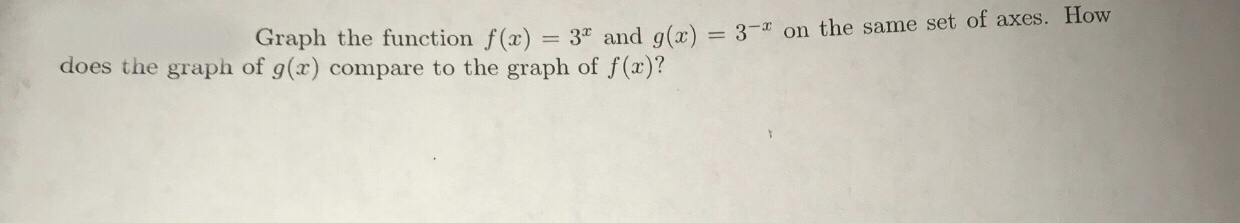# Graph the function f(x)3° and g(x)-3-x on the same set of axes. Howdoes the graph of g(x) compare to the graph of f (a)?

Question
27 viewshelp_outlineImage TranscriptioncloseGraph the function f(x) 3° and g(x)-3-x on the same set of axes. How does the graph of g(x) compare to the graph of f (a)? fullscreen
check_circle

star
star
star
star
star
1 Rating
Step 1

To draw the graph of the function f(x) = 3x, find the value of the function at different points to form ordered pairs. So, some ordered pairs are (-2, 0.11), (-1, 0.33), (0,1), (1 ,3), (2,9).

Step 2

Plot the ordered pairs on a graph and join them with a free hand. Also, as x goes to infinity, the function approaches infinity as well. So, the function will keep on increasing as we increase the value of x.

Step 3

As x goes to negative infinity, the function approaches 0. Also, the graph will not touch the x-axis as the function i...

### Want to see the full answer?

See Solution

#### Want to see this answer and more?

Solutions are written by subject experts who are available 24/7. Questions are typically answered within 1 hour.*

See Solution
*Response times may vary by subject and question.
Tagged in

### Calculus